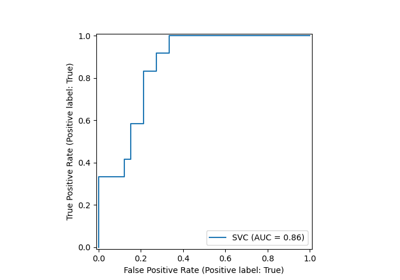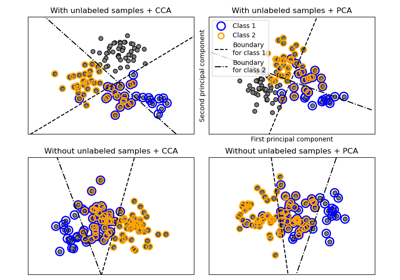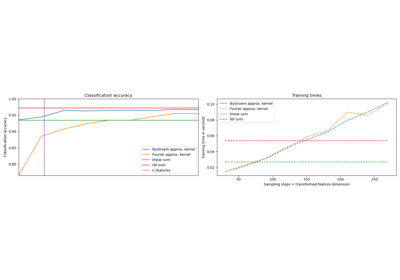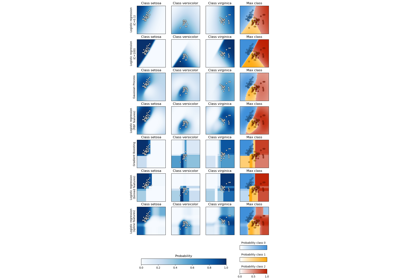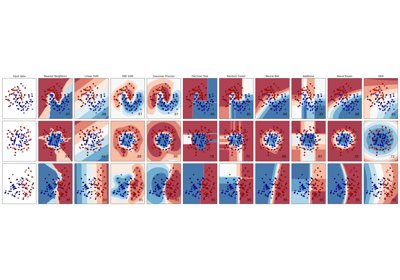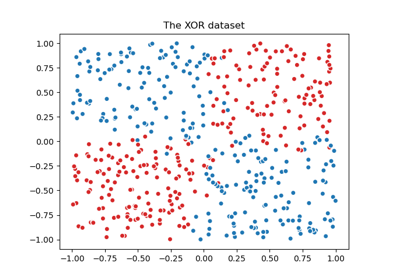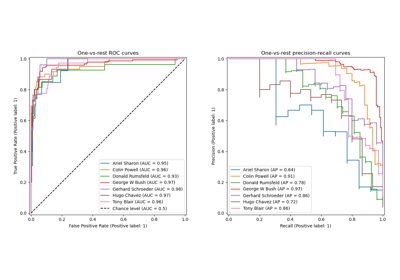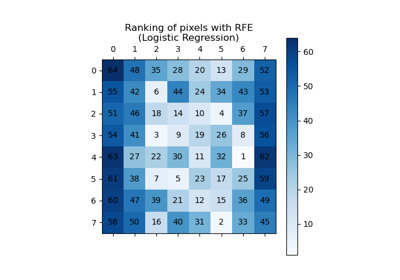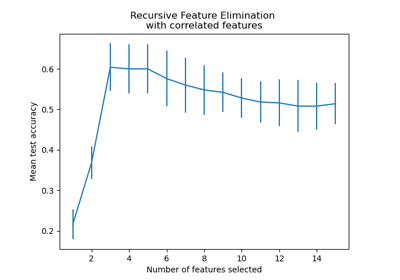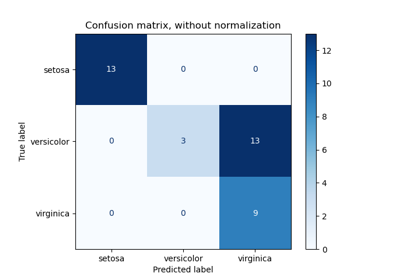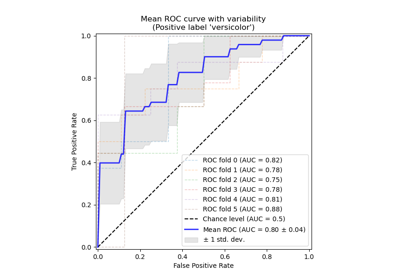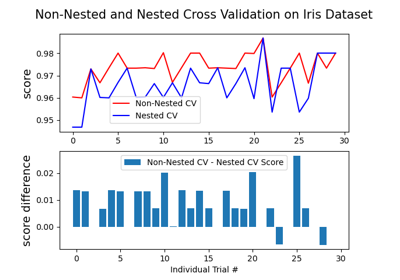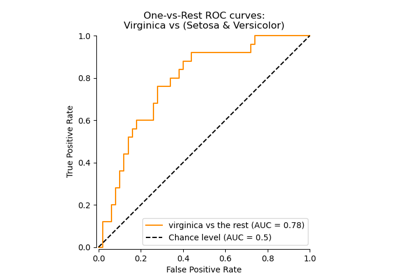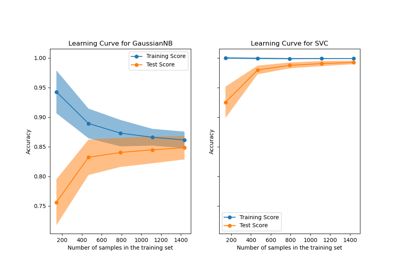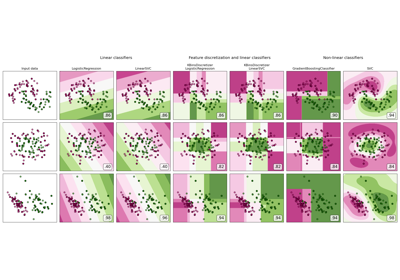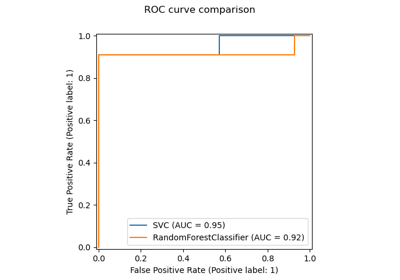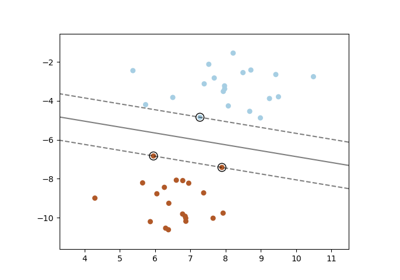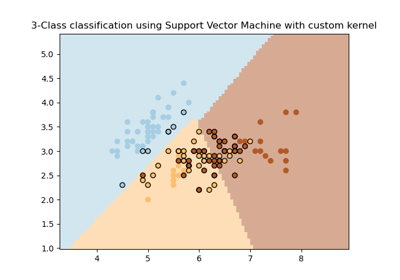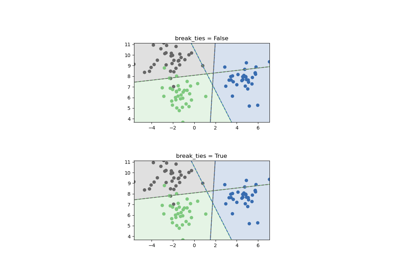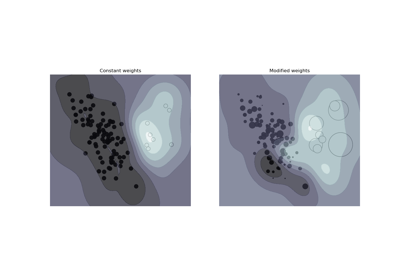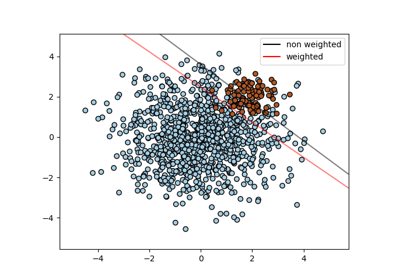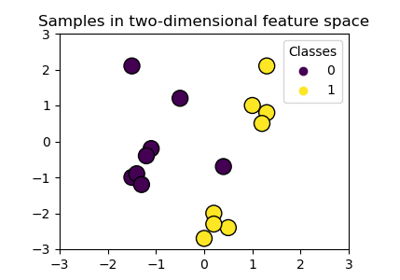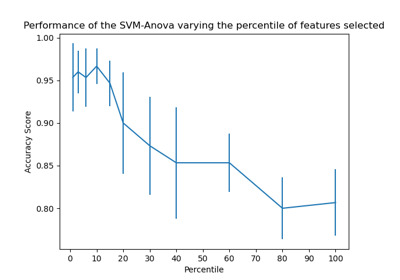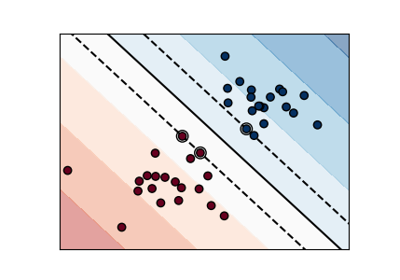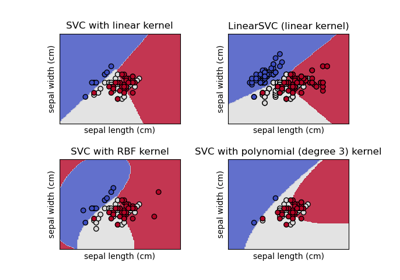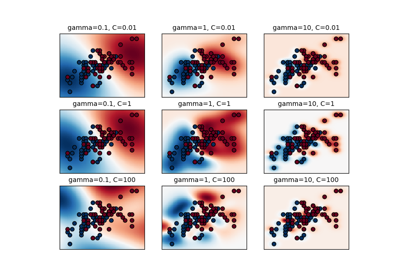# sklearn.svm.SVC¶

class sklearn.svm.SVC(C=1.0, kernel='rbf', degree=3, gamma='scale', coef0=0.0, shrinking=True, probability=False, tol=0.001, cache_size=200, class_weight=None, verbose=False, max_iter=-1, decision_function_shape='ovr', break_ties=False, random_state=None)[source]

C-Support Vector Classification.

The implementation is based on libsvm. The fit time scales at least quadratically with the number of samples and may be impractical beyond tens of thousands of samples. For large datasets consider using sklearn.svm.LinearSVC or sklearn.linear_model.SGDClassifier instead, possibly after a sklearn.kernel_approximation.Nystroem transformer.

The multiclass support is handled according to a one-vs-one scheme.

For details on the precise mathematical formulation of the provided kernel functions and how gamma, coef0 and degree affect each other, see the corresponding section in the narrative documentation: Kernel functions.

Read more in the User Guide.

Parameters
Cfloat, optional (default=1.0)

Regularization parameter. The strength of the regularization is inversely proportional to C. Must be strictly positive. The penalty is a squared l2 penalty.

kernelstring, optional (default=’rbf’)

Specifies the kernel type to be used in the algorithm. It must be one of ‘linear’, ‘poly’, ‘rbf’, ‘sigmoid’, ‘precomputed’ or a callable. If none is given, ‘rbf’ will be used. If a callable is given it is used to pre-compute the kernel matrix from data matrices; that matrix should be an array of shape (n_samples, n_samples).

degreeint, optional (default=3)

Degree of the polynomial kernel function (‘poly’). Ignored by all other kernels.

gamma{‘scale’, ‘auto’} or float, optional (default=’scale’)

Kernel coefficient for ‘rbf’, ‘poly’ and ‘sigmoid’.

• if gamma='scale' (default) is passed then it uses 1 / (n_features * X.var()) as value of gamma,

• if ‘auto’, uses 1 / n_features.

Changed in version 0.22: The default value of gamma changed from ‘auto’ to ‘scale’.

coef0float, optional (default=0.0)

Independent term in kernel function. It is only significant in ‘poly’ and ‘sigmoid’.

shrinkingboolean, optional (default=True)

Whether to use the shrinking heuristic.

probabilityboolean, optional (default=False)

Whether to enable probability estimates. This must be enabled prior to calling fit, will slow down that method as it internally uses 5-fold cross-validation, and predict_proba may be inconsistent with predict. Read more in the User Guide.

tolfloat, optional (default=1e-3)

Tolerance for stopping criterion.

cache_sizefloat, optional

Specify the size of the kernel cache (in MB).

class_weight{dict, ‘balanced’}, optional

Set the parameter C of class i to class_weight[i]*C for SVC. If not given, all classes are supposed to have weight one. The “balanced” mode uses the values of y to automatically adjust weights inversely proportional to class frequencies in the input data as n_samples / (n_classes * np.bincount(y))

verbosebool, default: False

Enable verbose output. Note that this setting takes advantage of a per-process runtime setting in libsvm that, if enabled, may not work properly in a multithreaded context.

max_iterint, optional (default=-1)

Hard limit on iterations within solver, or -1 for no limit.

decision_function_shape‘ovo’, ‘ovr’, default=’ovr’

Whether to return a one-vs-rest (‘ovr’) decision function of shape (n_samples, n_classes) as all other classifiers, or the original one-vs-one (‘ovo’) decision function of libsvm which has shape (n_samples, n_classes * (n_classes - 1) / 2). However, one-vs-one (‘ovo’) is always used as multi-class strategy.

Changed in version 0.19: decision_function_shape is ‘ovr’ by default.

New in version 0.17: decision_function_shape=’ovr’ is recommended.

Changed in version 0.17: Deprecated decision_function_shape=’ovo’ and None.

break_tiesbool, optional (default=False)

If true, decision_function_shape='ovr', and number of classes > 2, predict will break ties according to the confidence values of decision_function; otherwise the first class among the tied classes is returned. Please note that breaking ties comes at a relatively high computational cost compared to a simple predict.

New in version 0.22.

random_stateint, RandomState instance or None, optional (default=None)

The seed of the pseudo random number generator used when shuffling the data for probability estimates. If int, random_state is the seed used by the random number generator; If RandomState instance, random_state is the random number generator; If None, the random number generator is the RandomState instance used by np.random.

Attributes
support_array-like of shape (n_SV)

Indices of support vectors.

support_vectors_array-like of shape (n_SV, n_features)

Support vectors.

n_support_array-like, dtype=int32, shape = [n_class]

Number of support vectors for each class.

dual_coef_array, shape = [n_class-1, n_SV]

Coefficients of the support vector in the decision function. For multiclass, coefficient for all 1-vs-1 classifiers. The layout of the coefficients in the multiclass case is somewhat non-trivial. See the section about multi-class classification in the SVM section of the User Guide for details.

coef_array, shape = [n_class * (n_class-1) / 2, n_features]

Weights assigned to the features (coefficients in the primal problem). This is only available in the case of a linear kernel.

coef_ is a readonly property derived from dual_coef_ and support_vectors_.

intercept_ndarray of shape (n_class * (n_class-1) / 2,)

Constants in decision function.

fit_status_int

0 if correctly fitted, 1 otherwise (will raise warning)

classes_array of shape (n_classes,)

The classes labels.

probA_array, shape = [n_class * (n_class-1) / 2]
probB_array, shape = [n_class * (n_class-1) / 2]

If probability=True, it corresponds to the parameters learned in Platt scaling to produce probability estimates from decision values. If probability=False, it’s an empty array. Platt scaling uses the logistic function 1 / (1 + exp(decision_value * probA_ + probB_)) where probA_ and probB_ are learned from the dataset [R20c70293ef72-2]. For more information on the multiclass case and training procedure see section 8 of [R20c70293ef72-1].

class_weight_ndarray of shape (n_class,)

Multipliers of parameter C for each class. Computed based on the class_weight parameter.

shape_fit_tuple of int of shape (n_dimensions_of_X,)

Array dimensions of training vector X.

SVR

Support Vector Machine for Regression implemented using libsvm.

LinearSVC

Scalable Linear Support Vector Machine for classification implemented using liblinear. Check the See also section of LinearSVC for more comparison element.

References

R20c70293ef72-1

LIBSVM: A Library for Support Vector Machines

R20c70293ef72-2

Platt, John (1999). “Probabilistic outputs for support vector machines and comparison to regularizedlikelihood methods.”

Examples

>>> import numpy as np
>>> X = np.array([[-1, -1], [-2, -1], [1, 1], [2, 1]])
>>> y = np.array([1, 1, 2, 2])
>>> from sklearn.svm import SVC
>>> clf = SVC(gamma='auto')
>>> clf.fit(X, y)
SVC(gamma='auto')
>>> print(clf.predict([[-0.8, -1]]))



Methods

 decision_function(self, X) Evaluates the decision function for the samples in X. fit(self, X, y[, sample_weight]) Fit the SVM model according to the given training data. get_params(self[, deep]) Get parameters for this estimator. predict(self, X) Perform classification on samples in X. score(self, X, y[, sample_weight]) Return the mean accuracy on the given test data and labels. set_params(self, \*\*params) Set the parameters of this estimator.
__init__(self, C=1.0, kernel='rbf', degree=3, gamma='scale', coef0=0.0, shrinking=True, probability=False, tol=0.001, cache_size=200, class_weight=None, verbose=False, max_iter=-1, decision_function_shape='ovr', break_ties=False, random_state=None)[source]

Initialize self. See help(type(self)) for accurate signature.

decision_function(self, X)[source]

Evaluates the decision function for the samples in X.

Parameters
Xarray-like, shape (n_samples, n_features)
Returns
Xarray-like, shape (n_samples, n_classes * (n_classes-1) / 2)

Returns the decision function of the sample for each class in the model. If decision_function_shape=’ovr’, the shape is (n_samples, n_classes).

Notes

If decision_function_shape=’ovo’, the function values are proportional to the distance of the samples X to the separating hyperplane. If the exact distances are required, divide the function values by the norm of the weight vector (coef_). See also this question for further details. If decision_function_shape=’ovr’, the decision function is a monotonic transformation of ovo decision function.

fit(self, X, y, sample_weight=None)[source]

Fit the SVM model according to the given training data.

Parameters
X{array-like, sparse matrix}, shape (n_samples, n_features)

Training vectors, where n_samples is the number of samples and n_features is the number of features. For kernel=”precomputed”, the expected shape of X is (n_samples, n_samples).

yarray-like, shape (n_samples,)

Target values (class labels in classification, real numbers in regression)

sample_weightarray-like, shape (n_samples,)

Per-sample weights. Rescale C per sample. Higher weights force the classifier to put more emphasis on these points.

Returns
selfobject

Notes

If X and y are not C-ordered and contiguous arrays of np.float64 and X is not a scipy.sparse.csr_matrix, X and/or y may be copied.

If X is a dense array, then the other methods will not support sparse matrices as input.

get_params(self, deep=True)[source]

Get parameters for this estimator.

Parameters
deepbool, default=True

If True, will return the parameters for this estimator and contained subobjects that are estimators.

Returns
paramsmapping of string to any

Parameter names mapped to their values.

predict(self, X)[source]

Perform classification on samples in X.

For an one-class model, +1 or -1 is returned.

Parameters
X{array-like, sparse matrix}, shape (n_samples, n_features)

For kernel=”precomputed”, the expected shape of X is [n_samples_test, n_samples_train]

Returns
y_predarray, shape (n_samples,)

Class labels for samples in X.

property predict_log_proba

Compute log probabilities of possible outcomes for samples in X.

The model need to have probability information computed at training time: fit with attribute probability set to True.

Parameters
Xarray-like, shape (n_samples, n_features)

For kernel=”precomputed”, the expected shape of X is [n_samples_test, n_samples_train]

Returns
Tarray-like, shape (n_samples, n_classes)

Returns the log-probabilities of the sample for each class in the model. The columns correspond to the classes in sorted order, as they appear in the attribute classes_.

Notes

The probability model is created using cross validation, so the results can be slightly different than those obtained by predict. Also, it will produce meaningless results on very small datasets.

property predict_proba

Compute probabilities of possible outcomes for samples in X.

The model need to have probability information computed at training time: fit with attribute probability set to True.

Parameters
Xarray-like, shape (n_samples, n_features)

For kernel=”precomputed”, the expected shape of X is [n_samples_test, n_samples_train]

Returns
Tarray-like, shape (n_samples, n_classes)

Returns the probability of the sample for each class in the model. The columns correspond to the classes in sorted order, as they appear in the attribute classes_.

Notes

The probability model is created using cross validation, so the results can be slightly different than those obtained by predict. Also, it will produce meaningless results on very small datasets.

score(self, X, y, sample_weight=None)[source]

Return the mean accuracy on the given test data and labels.

In multi-label classification, this is the subset accuracy which is a harsh metric since you require for each sample that each label set be correctly predicted.

Parameters
Xarray-like of shape (n_samples, n_features)

Test samples.

yarray-like of shape (n_samples,) or (n_samples, n_outputs)

True labels for X.

sample_weightarray-like of shape (n_samples,), default=None

Sample weights.

Returns
scorefloat

Mean accuracy of self.predict(X) wrt. y.

set_params(self, **params)[source]

Set the parameters of this estimator.

The method works on simple estimators as well as on nested objects (such as pipelines). The latter have parameters of the form <component>__<parameter> so that it’s possible to update each component of a nested object.

Parameters
**paramsdict

Estimator parameters.

Returns
selfobject

Estimator instance.

## Examples using sklearn.svm.SVC¶